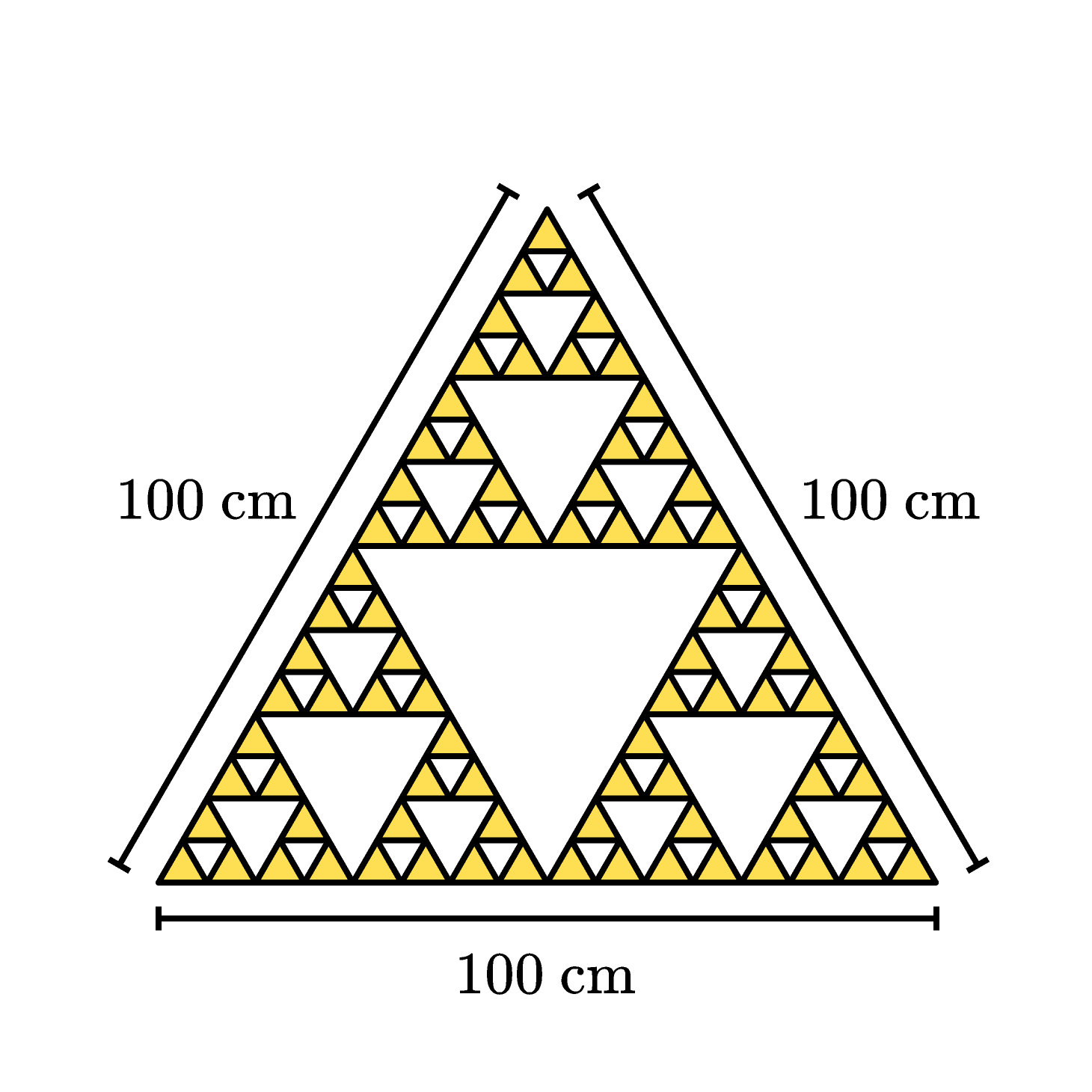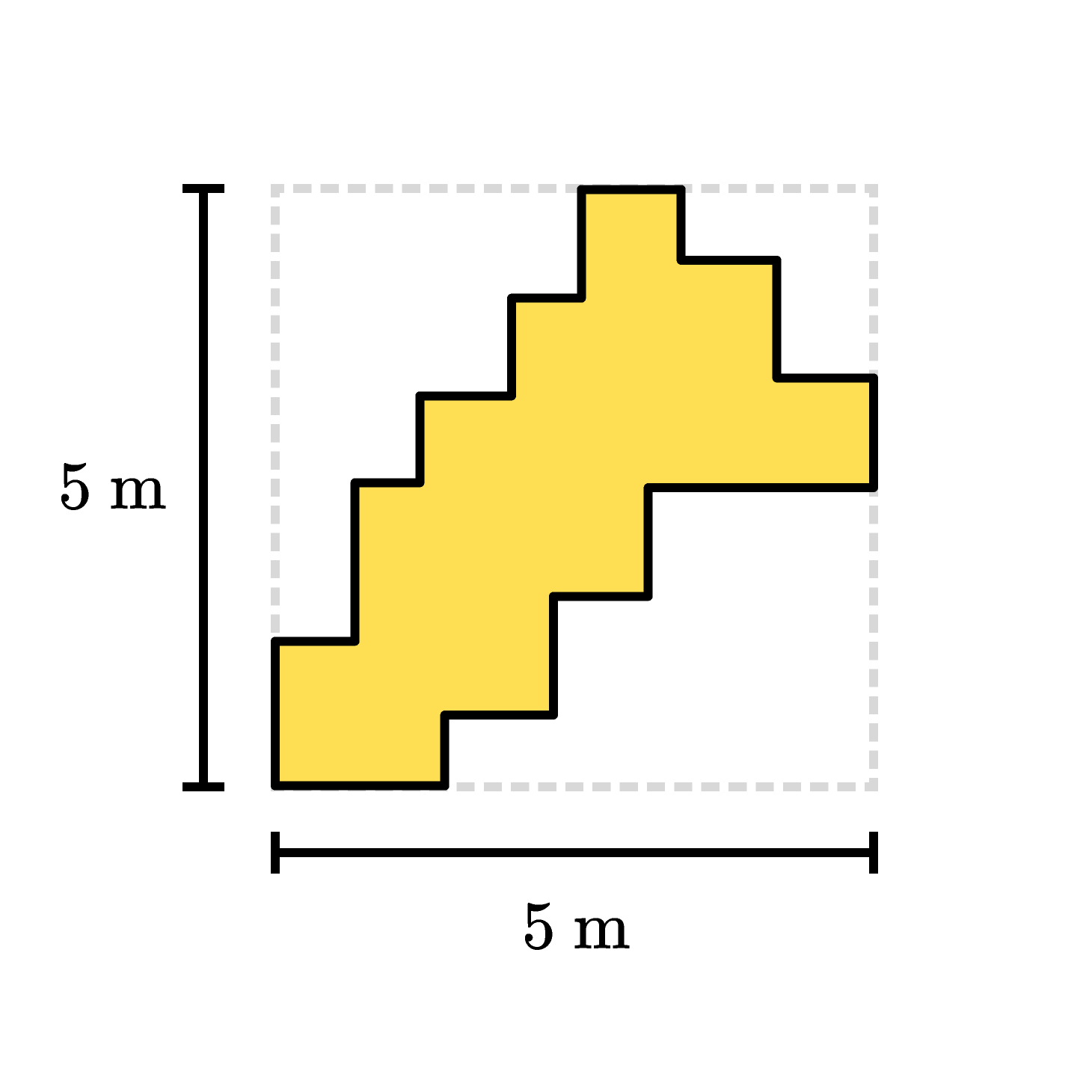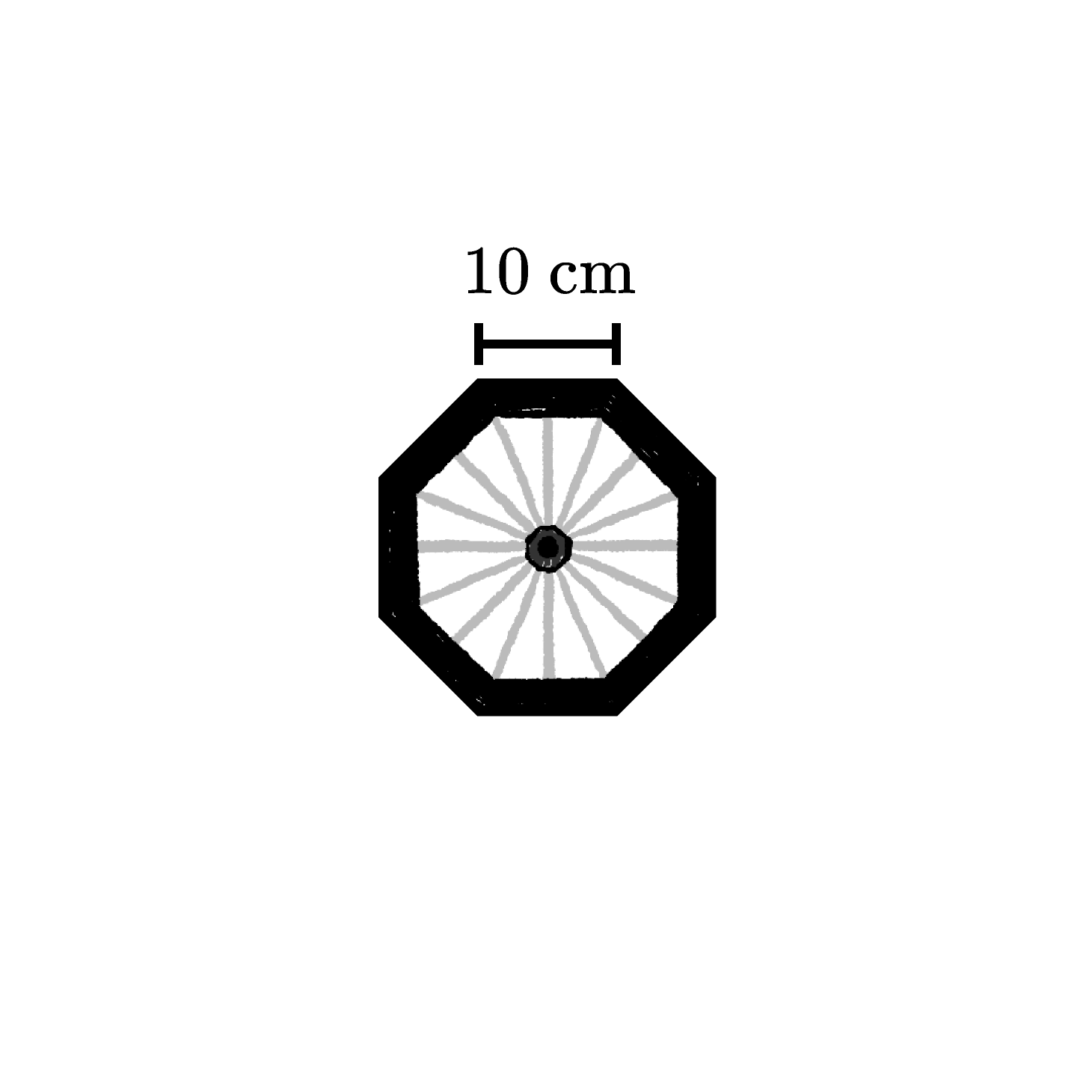### Geometry Fundamentals

Welcome to Geometry Fundamentals! In this course, you'll explore geometry and master challenging geometric problem-solving techniques.

There won't be a lot of formulas. Instead, you'll focus on strengthening your foundational geometric reasoning skills and intuition.

Memorizing formulas is a poor substitute for learning to use your own reasoning and intuition.

# Exploring Length

This course will cover a lot of ground. After reviewing some basics...

...the techniques in this course will quickly diversify. You'll need to use strategic, mathematical thinking, not a ruler, to solve each challenge.

And instead of memorizing a formula to calculate the lengths and areas of just the simplest shapes, you'll build intuition for how to simplify shapes you've never seen before...

...and then more easily calculate that shape's measurements.

# Exploring Length

If each side of both the triangle and regular hexagon is $3 \text{ cm}$ long, what is the longest distance across the hexagon?

The sliders below the figure let you move the triangle.

# Exploring LengthEvery small, shaded triangle in the design above is the same size. What is the side length of one of the small, shaded triangles?

# Exploring Length

All line segments in the shaded shape below are horizontal or vertical, and it's been drawn inside a $5 \text{ m}$ by $5 \text{ m}$ square. What is the distance around all the edges, or perimeter, of the shaded shape?# Exploring Length

Each of these three rectangles measures $3 \text{ cm}$ by $1 \text{ cm,}$ and the sliders below the figure let you move two of the rectangles.

Join the three rectangles into a single figure using the motions that the sliders allow, to make a shape with the smallest possible perimeter. What is the length of that perimeter?

# Exploring Length

The wheel below is in the shape of a regular octagon. If it rolled one complete turn across flat, level ground, how far would it go?# Exploring Length

The distance completely around each of the nine circles in the design below is $4 \text{ m}.$ What is the total length of the dark, meandering path in the figure?×